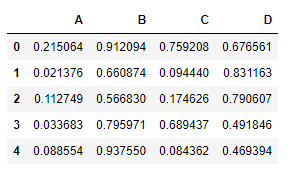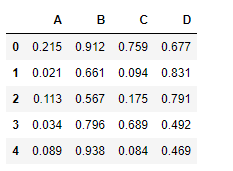Open in App
Not now

# Python | Pandas dataframe.round()

• Difficulty Level : Basic
• Last Updated : 30 Aug, 2021

Python is a great language for doing data analysis, primarily because of the fantastic ecosystem of data-centric python packages. Pandas is one of those packages and makes importing and analyzing data much easier.

Pandas` dataframe.round()` function is used to round a DataFrame to a variable number of decimal places. This function provides the flexibility to round different columns by different places.

Syntax:DataFrame.round(decimals=0, *args, **kwargs)
Parameters :
decimals : Number of decimal places to round each column to. If an int is given, round each column to the same number of places. Otherwise dict and Series round to variable numbers of places. Column names should be in the keys if decimals is a dict-like, or in the index if decimals is a Series. Any columns not included in decimals will be left as is. Elements of decimals which are not columns of the input will be ignored.

Returns : DataFrame object

Example #1: Use `round()` function to round off all columns in the dataframe to 3 decimal places

Note : We need to populate our dataframe with decimal values. Let’s use numpy random function to achieve the task.

 `# importing pandas as pd ` `import` `pandas as pd ` ` `  `# importing numpy as np ` `import` `numpy as np ` ` `  `# setting the seed to re-create the dataframe ` `np.random.seed(``25``) ` ` `  `# Creating a 5 * 4 dataframe  ` `df ``=` `pd.DataFrame(np.random.random([``5``, ``4``]), columns ``=``[``"A"``, ``"B"``, ``"C"``, ``"D"``]) ` ` `  `# Print the dataframe ` `df `Lets use the `dataframe.round()` function to round off all the decimal values in the dataframe to 3 decimal places.

 `df.``round``(``3``) `

Output :Example #2: Use `round()` function to round off all the columns in dataframe to different places.

 `# importing pandas as pd ` `import` `pandas as pd ` ` `  `# importing numpy as np ` `import` `numpy as np ` ` `  `# setting the seed to re-create the dataframe ` `np.random.seed(``25``) ` ` `  `# Creating a 5 * 4 dataframe  ` `df ``=` `pd.DataFrame(np.random.random([``5``, ``4``]), columns ``=``[``"A"``, ``"B"``, ``"C"``, ``"D"``]) ` ` `  `# Print the dataframe ` `df `Lets perform round off each column to different places

 `# round off the columns in this manner ` `# "A" to 1 decimal place ` `# "B" to 2 decimal place ` `# "C" to 3 decimal place ` `# "D" to 4 decimal place ` ` `  `df.``round``({``"A"``:``1``, ``"B"``:``2``, ``"C"``:``3``, ``"D"``:``4``}) `

Output :My Personal Notes arrow_drop_up
Related Articles